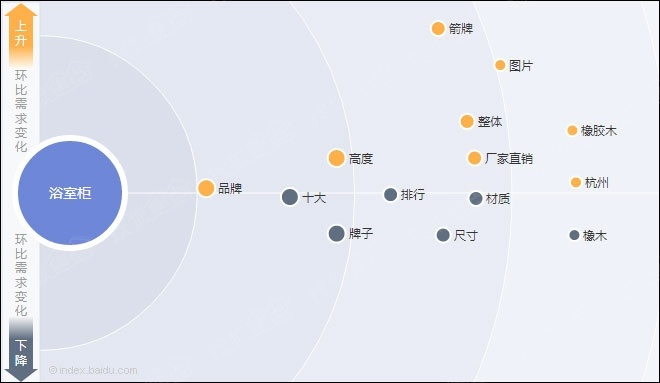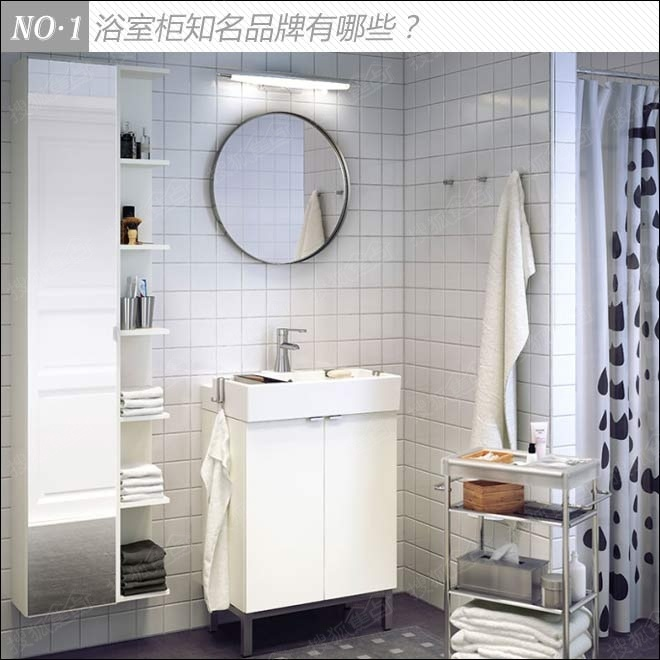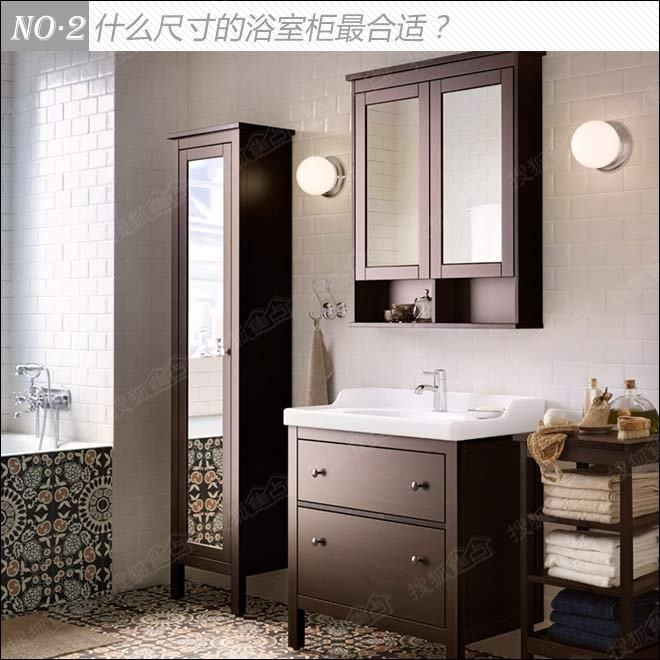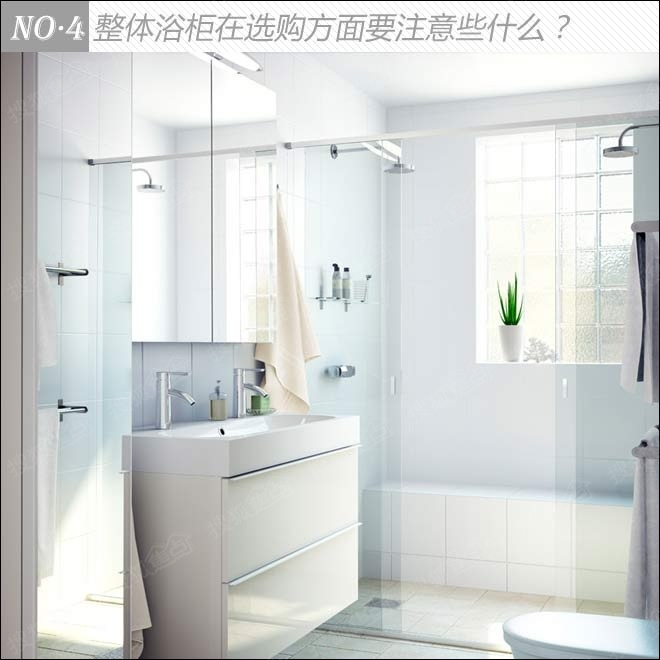|

# 你问我答 这些选购浴室柜的常识全部被我承包了

选购浴室柜你必须了解的事情

市面上的浴室柜款式众多，琳琅满目，到底应该把哪款带回家呢？浴室柜的十大品牌有哪些呢？哪些品牌靠谱一点呢？在安装浴室柜时要注意些什么呢？浴室柜有标准的尺寸吗？那种材质的浴室柜好啊？在选择时，这些问题让消费者抓破脑袋，别烦恼啦，下面编辑为你一一解答。看图说话：

数据来源于百度指数，时间段为2013年8月至2014年8月。从数据中读懂网友需求。1、与大部分的家具一样，在选购浴室柜时人们最关心的始终是品牌。有口碑、有实力的卫浴品牌是消费者们优先考虑的对象。在品牌中我们可以看到其中有一个品牌“箭牌”得到比较多的关注，除“箭牌”外，市面上有哪些比较知名的卫浴品牌呢？

2、从图中我们可以看到，在浴室柜的选择方面，人们更加关心的是浴室柜的高度，到底怎样的浴室柜高度最合适呢？在选择浴室柜时在尺寸方面有哪些需要注意的呢？

3、浴室柜的材质也是人们关心的问题之一，浴室柜有哪些材质呢？选择什么材质最实用呢？

4、在选购浴室柜时，整体浴室柜更受消费者的欢迎，消费者更加注重卫浴间的整体搭配设计。

你问我答：

浴室柜知名品牌有哪些？浴室柜十大品牌有哪些？目前市面上比较知名的浴室柜品牌分别是箭牌浴室柜、安华浴室柜、科勒浴室柜、 法恩莎浴室柜、英皇CRW浴室柜、心海伽蓝浴室柜、恒洁浴室柜、尚高浴室柜、朗司浴室柜、欧路莎浴室柜。这些品牌大多来自广东佛山，是目前是市面上比较畅销的品牌之一。

市场上的产品品牌种类繁多，消费者以些作为选购前的参考资料，是一种不错的方式之一。

浴室柜如何安装合适的高度尺寸？对于浴室柜的安装，更多的网友关心的是浴室柜的高度。对于浴室柜的高度，其实并没有严格的要求，主要是根据主人的身高调整。一般浴室柜的安装尺寸是，柜子表面离地面的距离是80mm～85mm。从地面到盆的上边缘80cm到85cm左右。

由于浴室的大小基本差不多，大部分浴室柜最常见的标准尺寸是长为800mm～1000mm，宽为450mm～500mm。对于超大的浴室柜和超小的浴室柜市场上都不多，但也可以根据实际要求特别定制。

浴室柜有哪些材质呢？选择什么材质最实用呢？在选购浴室柜时，关键是板材的防水功能。为了让板材具有良好的防水功能，一般会在板材表面做烤漆处理，尽量确保板材的密封性，使水汽尽量少的与板材直接接触。因此，我们在挑选浴室柜时，最重要的就是柜体的板材。目前浴室柜的材质主要有实木板材、PVC材质、橡木材质、不锈钢材质、陶瓷材质以及玻璃材质等。而比较常见的就是PVC材质、橡木板材以及其他实木板材。

pvc板材防水性能优越，而且价格比较低廉，但是不上档次。实木板材表面经过处理后，吸水率可下降到5%之内。看上去虽然上档次，整体美观大方，但是价格相对来说比较贵。其中，橡木材质的实木浴室柜在市面上比较受到青睐，质地坚硬，色泽鲜艳；并且耐变形、防潮性能好。

整体浴柜在选购方面要注意些什么？在选购浴室柜时，要注意以下四点：

1、对普通家庭来说，浴室柜最好选择挂墙式、柜腿较高或是带轮子的，这样可以有效地隔离地面潮气。

2、要了解所有金属件是否是经过防潮处理的不锈钢或浴柜专用的铝制品，这样抗湿性能才会有保障。

3、有必要检查浴室柜合页的开启度。开启角度达到180度时，可以更方便地取放物品。

4、在挑选浴柜款式时，要保障进出水管的检修和阀门的开启，不要给以后的维护和检修留下麻烦。

5、考虑卫浴间面积，大空间要大而得当，小空间要各项功能俱全。

在卫浴空间相对较大的情况下，最好做到干湿分离，淋浴间和其他区域相分隔，保证淋浴的水不会四处飞溅，使淋浴以外的空间保持干燥。在选择浴室柜时，才有更多的选择，同时可以延长浴室柜的使用寿命。

如果卫浴间实在太小了，只有四五平方左右，难以实现干湿分离。这种情况下可选购吊挂在墙角的或是离地面较高的浴柜，这样往上层空间发展，也可以有效地利用起小空间。

`声明：本文由入驻焦点开放平台的作者撰写，除焦点官方账号外，观点仅代表作者本人，不代表焦点立场错误信息举报电话： 400-099-0099，邮箱：jubao@vip.sohu.com，或点此进行意见反馈，或点此进行举报投诉。`A B C D E F G H J K L M N P Q R S T W X Y Z
A - B - C - D - E
• A
• 鞍山
• 安庆
• 安阳
• 安顺
• 安康
• 澳门
• B
• 北京
• 保定
• 包头
• 巴彦淖尔
• 本溪
• 蚌埠
• 亳州
• 滨州
• 北海
• 百色
• 巴中
• 毕节
• 保山
• 宝鸡
• 白银
• 巴州
• C
• 承德
• 沧州
• 长治
• 赤峰
• 朝阳
• 长春
• 常州
• 滁州
• 池州
• 长沙
• 常德
• 郴州
• 潮州
• 崇左
• 重庆
• 成都
• 楚雄
• 昌都
• 慈溪
• 常熟
• D
• 大同
• 大连
• 丹东
• 大庆
• 东营
• 德州
• 东莞
• 德阳
• 达州
• 大理
• 德宏
• 定西
• 儋州
• 东平
• E
• 鄂尔多斯
• 鄂州
• 恩施
F - G - H - I - J
• F
• 抚顺
• 阜新
• 阜阳
• 福州
• 抚州
• 佛山
• 防城港
• G
• 赣州
• 广州
• 桂林
• 贵港
• 广元
• 广安
• 贵阳
• 固原
• H
• 邯郸
• 衡水
• 呼和浩特
• 呼伦贝尔
• 葫芦岛
• 哈尔滨
• 黑河
• 淮安
• 杭州
• 湖州
• 合肥
• 淮南
• 淮北
• 黄山
• 菏泽
• 鹤壁
• 黄石
• 黄冈
• 衡阳
• 怀化
• 惠州
• 河源
• 贺州
• 河池
• 海口
• 红河
• 汉中
• 海东
• 怀来
• I
• J
• 晋中
• 锦州
• 吉林
• 鸡西
• 佳木斯
• 嘉兴
• 金华
• 景德镇
• 九江
• 吉安
• 济南
• 济宁
• 焦作
• 荆门
• 荆州
• 江门
• 揭阳
• 金昌
• 酒泉
• 嘉峪关
K - L - M - N - P
• K
• 开封
• 昆明
• 昆山
• L
• 廊坊
• 临汾
• 辽阳
• 连云港
• 丽水
• 六安
• 龙岩
• 莱芜
• 临沂
• 聊城
• 洛阳
• 漯河
• 娄底
• 柳州
• 来宾
• 泸州
• 乐山
• 六盘水
• 丽江
• 临沧
• 拉萨
• 林芝
• 兰州
• 陇南
• M
• 牡丹江
• 马鞍山
• 茂名
• 梅州
• 绵阳
• 眉山
• N
• 南京
• 南通
• 宁波
• 南平
• 宁德
• 南昌
• 南阳
• 南宁
• 内江
• 南充
• P
• 盘锦
• 莆田
• 平顶山
• 濮阳
• 攀枝花
• 普洱
• 平凉
Q - R - S - T - W
• Q
• 秦皇岛
• 齐齐哈尔
• 衢州
• 泉州
• 青岛
• 清远
• 钦州
• 黔南
• 曲靖
• 庆阳
• R
• 日照
• 日喀则
• S
• 石家庄
• 沈阳
• 双鸭山
• 绥化
• 上海
• 苏州
• 宿迁
• 绍兴
• 宿州
• 三明
• 上饶
• 三门峡
• 商丘
• 十堰
• 随州
• 邵阳
• 韶关
• 深圳
• 汕头
• 汕尾
• 三亚
• 三沙
• 遂宁
• 山南
• 商洛
• 石嘴山
• T
• 天津
• 唐山
• 太原
• 通辽
• 铁岭
• 泰州
• 台州
• 铜陵
• 泰安
• 铜仁
• 铜川
• 天水
• 天门
• W
• 乌海
• 乌兰察布
• 无锡
• 温州
• 芜湖
• 潍坊
• 威海
• 武汉
• 梧州
• 渭南
• 武威
• 吴忠
• 乌鲁木齐
X - Y - Z
• X
• 邢台
• 徐州
• 宣城
• 厦门
• 新乡
• 许昌
• 信阳
• 襄阳
• 孝感
• 咸宁
• 湘潭
• 湘西
• 西双版纳
• 西安
• 咸阳
• 西宁
• 仙桃
• 西昌
• Y
• 运城
• 营口
• 盐城
• 扬州
• 鹰潭
• 宜春
• 烟台
• 宜昌
• 岳阳
• 益阳
• 永州
• 阳江
• 云浮
• 玉林
• 宜宾
• 雅安
• 玉溪
• 延安
• 榆林
• 银川
• Z
• 张家口
• 镇江
• 舟山
• 漳州
• 淄博
• 枣庄
• 郑州
• 周口
• 驻马店
• 株洲
• 张家界
• 珠海
• 湛江
• 肇庆
• 中山
• 自贡
• 资阳
• 遵义
• 昭通
• 张掖
• 中卫

1室1厅1厨1卫1阳台

1
2
3
4
5

0
1
2

1

1

0
1
2
3报名成功，资料已提交审核A B C D E F G H J K L M N P Q R S T W X Y Z
A - B - C - D - E
• A
• 鞍山
• 安庆
• 安阳
• 安顺
• 安康
• 澳门
• B
• 北京
• 保定
• 包头
• 巴彦淖尔
• 本溪
• 蚌埠
• 亳州
• 滨州
• 北海
• 百色
• 巴中
• 毕节
• 保山
• 宝鸡
• 白银
• 巴州
• C
• 承德
• 沧州
• 长治
• 赤峰
• 朝阳
• 长春
• 常州
• 滁州
• 池州
• 长沙
• 常德
• 郴州
• 潮州
• 崇左
• 重庆
• 成都
• 楚雄
• 昌都
• 慈溪
• 常熟
• D
• 大同
• 大连
• 丹东
• 大庆
• 东营
• 德州
• 东莞
• 德阳
• 达州
• 大理
• 德宏
• 定西
• 儋州
• 东平
• E
• 鄂尔多斯
• 鄂州
• 恩施
F - G - H - I - J
• F
• 抚顺
• 阜新
• 阜阳
• 福州
• 抚州
• 佛山
• 防城港
• G
• 赣州
• 广州
• 桂林
• 贵港
• 广元
• 广安
• 贵阳
• 固原
• H
• 邯郸
• 衡水
• 呼和浩特
• 呼伦贝尔
• 葫芦岛
• 哈尔滨
• 黑河
• 淮安
• 杭州
• 湖州
• 合肥
• 淮南
• 淮北
• 黄山
• 菏泽
• 鹤壁
• 黄石
• 黄冈
• 衡阳
• 怀化
• 惠州
• 河源
• 贺州
• 河池
• 海口
• 红河
• 汉中
• 海东
• 怀来
• I
• J
• 晋中
• 锦州
• 吉林
• 鸡西
• 佳木斯
• 嘉兴
• 金华
• 景德镇
• 九江
• 吉安
• 济南
• 济宁
• 焦作
• 荆门
• 荆州
• 江门
• 揭阳
• 金昌
• 酒泉
• 嘉峪关
K - L - M - N - P
• K
• 开封
• 昆明
• 昆山
• L
• 廊坊
• 临汾
• 辽阳
• 连云港
• 丽水
• 六安
• 龙岩
• 莱芜
• 临沂
• 聊城
• 洛阳
• 漯河
• 娄底
• 柳州
• 来宾
• 泸州
• 乐山
• 六盘水
• 丽江
• 临沧
• 拉萨
• 林芝
• 兰州
• 陇南
• M
• 牡丹江
• 马鞍山
• 茂名
• 梅州
• 绵阳
• 眉山
• N
• 南京
• 南通
• 宁波
• 南平
• 宁德
• 南昌
• 南阳
• 南宁
• 内江
• 南充
• P
• 盘锦
• 莆田
• 平顶山
• 濮阳
• 攀枝花
• 普洱
• 平凉
Q - R - S - T - W
• Q
• 秦皇岛
• 齐齐哈尔
• 衢州
• 泉州
• 青岛
• 清远
• 钦州
• 黔南
• 曲靖
• 庆阳
• R
• 日照
• 日喀则
• S
• 石家庄
• 沈阳
• 双鸭山
• 绥化
• 上海
• 苏州
• 宿迁
• 绍兴
• 宿州
• 三明
• 上饶
• 三门峡
• 商丘
• 十堰
• 随州
• 邵阳
• 韶关
• 深圳
• 汕头
• 汕尾
• 三亚
• 三沙
• 遂宁
• 山南
• 商洛
• 石嘴山
• T
• 天津
• 唐山
• 太原
• 通辽
• 铁岭
• 泰州
• 台州
• 铜陵
• 泰安
• 铜仁
• 铜川
• 天水
• 天门
• W
• 乌海
• 乌兰察布
• 无锡
• 温州
• 芜湖
• 潍坊
• 威海
• 武汉
• 梧州
• 渭南
• 武威
• 吴忠
• 乌鲁木齐
X - Y - Z
• X
• 邢台
• 徐州
• 宣城
• 厦门
• 新乡
• 许昌
• 信阳
• 襄阳
• 孝感
• 咸宁
• 湘潭
• 湘西
• 西双版纳
• 西安
• 咸阳
• 西宁
• 仙桃
• 西昌
• Y
• 运城
• 营口
• 盐城
• 扬州
• 鹰潭
• 宜春
• 烟台
• 宜昌
• 岳阳
• 益阳
• 永州
• 阳江
• 云浮
• 玉林
• 宜宾
• 雅安
• 玉溪
• 延安
• 榆林
• 银川
• Z
• 张家口
• 镇江
• 舟山
• 漳州
• 淄博
• 枣庄
• 郑州
• 周口
• 驻马店
• 株洲
• 张家界
• 珠海
• 湛江
• 肇庆
• 中山
• 自贡
• 资阳
• 遵义
• 昭通
• 张掖
• 中卫• 手机• 分享
• 设计
免费设计
• 计算器
装修计算器
• 入驻
合作入驻
• 联系
联系我们
• 置顶
返回顶部